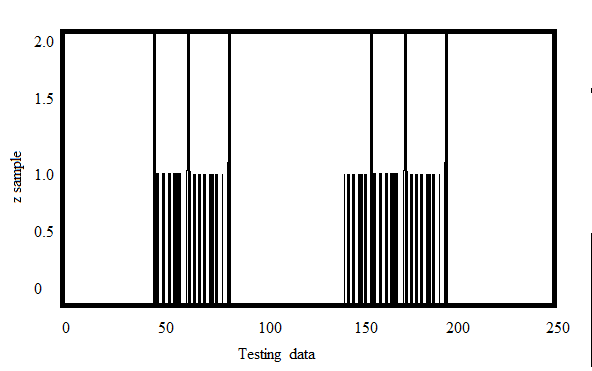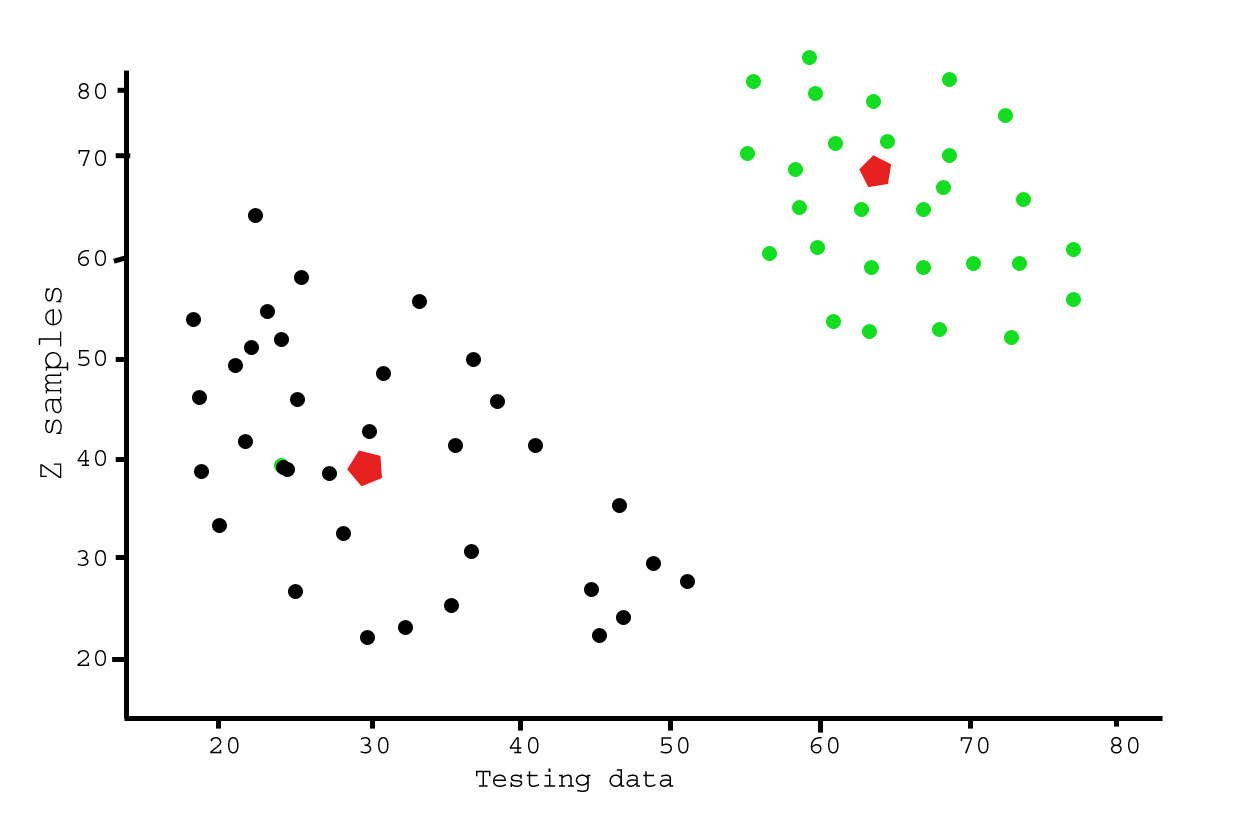# Analysis of test data using K-Means Clustering in Python

This article demonstrates an illustration of K-means clustering on a sample random data using open-cv library.

Pre-requisites: Numpy, OpenCV, matplot-lib
Let’s first visualize test data with Multiple Features using matplot-lib tool.

 `# importing required tools ` `import` `numpy as np ` `from` `matplotlib ``import` `pyplot as plt ` ` `  `# creating two test data ` `X ``=` `np.random.randint(``10``,``35``,(``25``,``2``)) ` `Y ``=` `np.random.randint(``55``,``70``,(``25``,``2``)) ` `Z ``=` `np.vstack((X,Y)) ` `Z ``=` `Z.reshape((``50``,``2``)) ` ` `  `# convert to np.float32 ` `Z ``=` `np.float32(Z) ` ` `  `plt.xlabel(``'Test Data'``) ` `plt.ylabel(``'Z samples'``) ` ` `  `plt.hist(Z,``256``,[``0``,``256``]) ` ` `  `plt.show() `

Here ‘Z’ is an array of size 100, and values ranging from 0 to 255. Now, reshaped ‘z’ to a column vector. It will be more useful when more than one features are present. Then change the data to np.float32 type.

Output:Now, apply the k-Means clustering algorithm to the same example as in the above test data and see its behavior.
Steps Involved:
1) First we need to set a test data.
2) Define criteria and apply kmeans().
3) Now separate the data.
4) Finally Plot the data.

 `import` `numpy as np ` `import` `cv2 ` `from` `matplotlib ``import` `pyplot as plt ` ` `  `X ``=` `np.random.randint(``10``,``45``,(``25``,``2``)) ` `Y ``=` `np.random.randint(``55``,``70``,(``25``,``2``)) ` `Z ``=` `np.vstack((X,Y)) ` ` `  `# convert to np.float32 ` `Z ``=` `np.float32(Z) ` ` `  `# define criteria and apply kmeans() ` `criteria ``=` `(cv2.TERM_CRITERIA_EPS ``+` `cv2.TERM_CRITERIA_MAX_ITER, ``10``, ``1.0``) ` `ret,label,center ``=` `cv2.kmeans(Z,``2``,``None``,criteria,``10``,cv2.KMEANS_RANDOM_CENTERS) ` ` `  `# Now separate the data ` `A ``=` `Z[label.ravel()``=``=``0``] ` `B ``=` `Z[label.ravel()``=``=``1``] ` ` `  `# Plot the data ` `plt.scatter(A[:,``0``],A[:,``1``]) ` `plt.scatter(B[:,``0``],B[:,``1``],c ``=` `'r'``) ` `plt.scatter(center[:,``0``],center[:,``1``],s ``=` `80``,c ``=` `'y'``, marker ``=` `'s'``) ` `plt.xlabel(``'Test Data'``),plt.ylabel(``'Z samples'``) ` `plt.show() `

Output:This example is meant to illustrate where k-means will produce intuitively possible clusters.

Applications:
1) Identifying Cancerous Data.
2) Prediction of Students’ Academic Performance.
3) Drug Activity Prediction.

Attention geek! Strengthen your foundations with the Python Programming Foundation Course and learn the basics.

To begin with, your interview preparations Enhance your Data Structures concepts with the Python DS Course.

My Personal Notes arrow_drop_upCheck out this Author's contributed articles.

If you like GeeksforGeeks and would like to contribute, you can also write an article using contribute.geeksforgeeks.org or mail your article to contribute@geeksforgeeks.org. See your article appearing on the GeeksforGeeks main page and help other Geeks.

Please Improve this article if you find anything incorrect by clicking on the "Improve Article" button below.